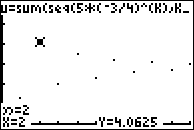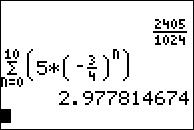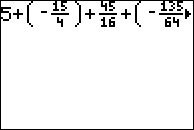••• ##### Device
• TI-83 Plus Family
• TI-84 Plus
• TI-84 Plus Silver Edition
•TI-84 Plus C Silver Edition
•TI-84 Plus CE

# Calculus: Exploring Infinite Series

by Texas Instruments#### Overview

In this activity, students learn to identify geometric series. They determine if a sequence converges and also learn to calculate the sum of numbers in a geometric series.

#### Key Steps

•Students will evaluate the convergence and sum of a geometric series.

•Students will identify a series that satisfies the alternating series test.

•Students will use the graphing calculator to approximate the sum of a series.